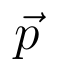Select Page

# Vector Algebra 12 Science Maths CBSE Solutions for MCQ in English

Vector Algebra 12 Science Maths CBSE Solutions for MCQ in English to enable students to get Solutions in a narrative video format for the specific question.

Expert Teacher provides Vector Algebra 12 Science Maths CBSE Solutions for MCQ through Video Solutions in English language. This video solution will be useful for students to understand how to write an answer in exam in order to score more marks. This teacher uses a narrative style for a question from Vector Algebra not only to explain the proper method of answering question, but deriving right answer too.

Please find the question below and view the Solution in a narrative video format.

Question:

Solution Video in English:

You can select video Solutions from other languages also. Please check Solutions in ( Hindi )

## Similar Questions from CBSE, 12th Science, Maths, Vector Algebra

Question 1 : Find the area of the parallelogram whose adjacent sides are determined by the vectorsand(View Answer Video)

Question 2 : Write the position vector of the point which divides the join of points with position vectorsandin the ratio 2:1.  (View Answer Video)

Question 3 : Find the angle between two vectors a and b with magnitudesand 2 respectively, having(View Answer Video)

Question 4 :  Write the value offor which the vectorsandare parallel vectors.  (View Answer Video)

Question 5 : Find the scalar quantities :
(i)     Work                     (ii)      Force

### Linear Programming

Question 1 : The objective function is maximum or minimum, which lies on the boundary of the feasible region. (View Answer Video)

### Continuity and Differentiability

Question 1 : Differentiate the functionwith respect to x. (View Answer Video)

Question 2 : Differentiate the function w.r.t.x. (View Answer Video)

Question 3 : Findfor the function. (View Answer Video)

Question 4 : Ifand, find. (View Answer Video)

Question 5 : Findfor the function. (View Answer Video)

### Three Dimensional Geometry

Question 1 : Find the cartesian equation of the line which passes through the point (-2, 4, -5) and is parallel to the line(View Answer Video)

Question 2 : Write the sum of intercepts cut off by the planeon the three axes.  (View Answer Video)

Question 3 : Find the vector equation of the line passing through the point (1, 2, 3) and parallel to the planesand(View Answer Video)

Question 4 : Find the equation of the plane with intercept 3 on the y-axis and parallel to ZOX plane. (View Answer Video)

Question 5 : Find the distance of the point (-1, -5, -10) from the point of intersection of the lineand the plane(View Answer Video)